Classification

Classification is defined as 'to assort the different items of a given group on the basis of certain common quality they possess and spot the odd man out.

Different type of questions covered in this chapter are as follows

1. Choosing the Odd Figure
2. Choosing Figure with Same Characteristics

This section 'Classification' covers several different criteria which can be applied to classify the given items into a particular group. This classification can be based on some common properties which they possess like shape, number of sides, division of figures etc. Here, the object which possess these common properties are classified into the same group while the object which does not possess these common properties (i.e., the odd one) is to be eliminated from the group.

Let us consider an example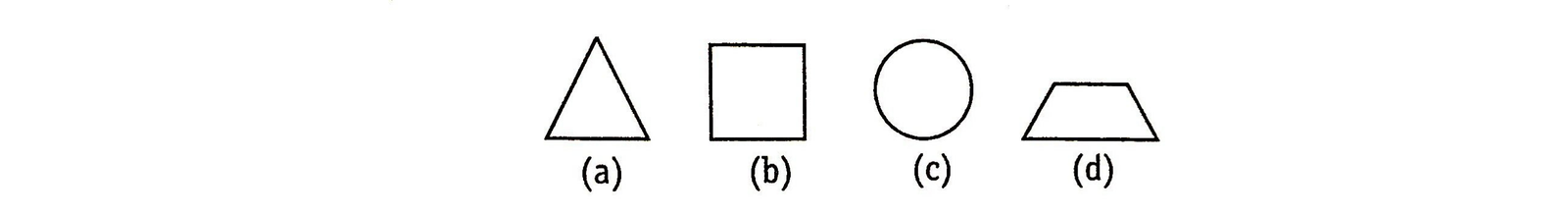In all the figures given above, except circle, all are formed with straight lines. Hence, circle does not fit into the group and is the odd one.

Several logics are applied for classification of given figures into a particular group based on some similarity pattern which is followed by all the figures of the group. This similarity pattern establishes a sort of equality between the figures and the figure which does not follow the similarity pattern (i.e., shows inequality) is to be eliminated from the group.

There are many criteria used for establishing equality-inequality between the figures which are given below

1. Rotation of Same Figure

This is the most common type of classification. The similar figures are actually the rotated forms of the same figure in clockwise or anti-clockwise direction. The figure which comes out to be different from other is that figure which cannot be obtained by rotation of either of the other figures.

Directions (Example Nos. 1-2) In the following questions, a group of five figures is given, Out of which four figures are similar to each other in a certain way and one is different from other. Find the odd figure out.

Example 1: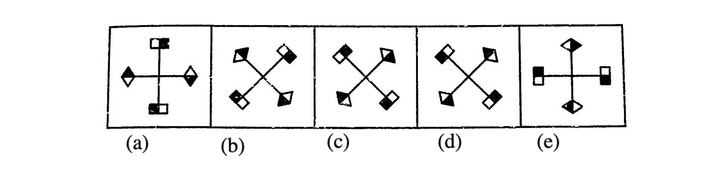Solution:  (d) After examining the above figure, it is found that except (d), all figures can easily be obtained by clockwise and anti-clockwise movement of each other.

Example 2: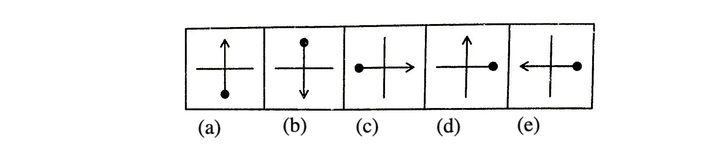Solution:  (d) All figure except figure (d), can be rotated to form different figures.

2. Number of Element or Lines

A group of figures may be classified on the basis of number of elements or the number of lines present in figures. The figures can also be classified on even or odd number or lines or elements present in figures. Classification can also be done on the ratio of number of lines and elements.

Directions (Example Nos. 1-4) In the following questions, a group of five figures is given, Out of which four figures are similar to each other in a certain way and one is different from other. Find the odd figure out.

Example 1: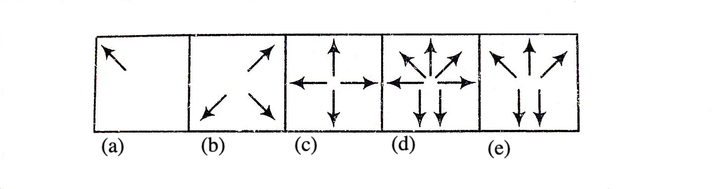Solution:  (c) All except, figure (c) contains odd number of arrows.

Example 2: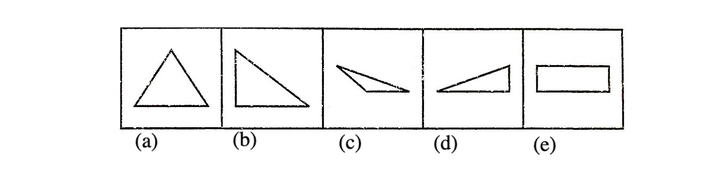Solution:  (e) Except figure (e), all have three sides but in figure (e) there are four sides.

Example 3: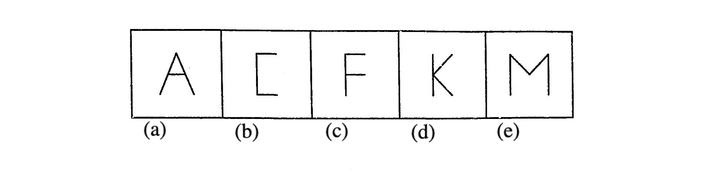Solution:  (e) Except figure (e), all figures are made-up of three simple lines but in option (e) four lines are involved.

Example 4: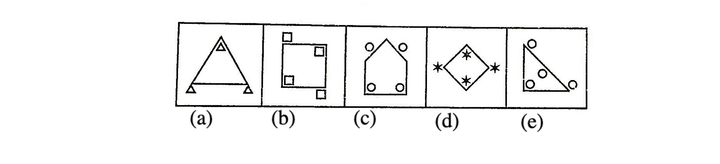Solution: (a) Except figure (a), all figures have equal number of figures both inside and outside of the main figure.

3. Division of Figures

This type of classification is done on the equal or inequal division of figures or division of figure in some specified ratio or parts.

Directions (Example Nos. 1-2) In the following questions, a group of five figures is given, Out of which four figures are similar to each other in a certain way and one is different from other. Find the odd figure out.

Example 1: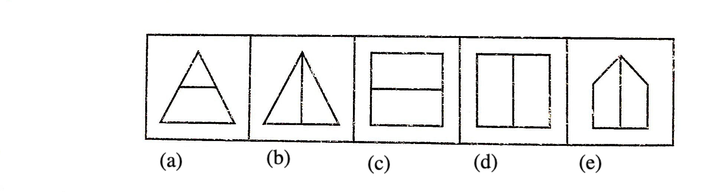Solution:  (a) Except figure (a), all figures are divided into two equal parts.

Example 2: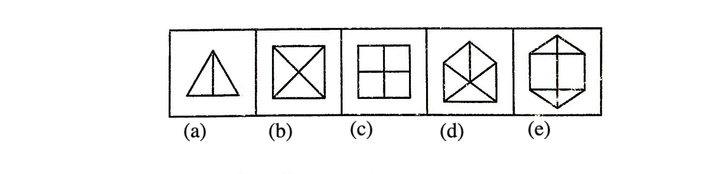Solution:  (a) Here each figure except (a) is divided such that each figure contains as many parts as equal to the number of sides in each figure.

4. Similarity of Figures

Classification on the basis of similarity of figure is done when orientation, shape, measure of angle or method of presentation of group is same except for the odd figure.

Directions (Example Nos. 1-2) In the following questions, a group of five figures is given, Out of which four figures are similar to each other in a certain way and one is different from other. Find the odd figure out.

Example 1: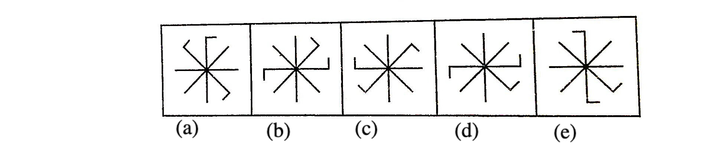Solution:  (d) Let us consider the two adjacent bent lines as a pair. Then, in each figure except (d), there are two straight lines between the bent pair and the remaining bent line when the direction of bent is considered.

Example 2: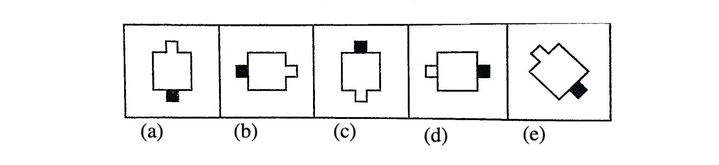Solution:  (d) Except figure (d), in all figures, middle of square is open that touches the blank box.

5. Relation between Elements of Figure

In this type of classification, the elements of the figure bears a certain relationship between them in which the odd figure does not posses. This relation can e based on shape of elements presents, inversion of elements etc.

Directions (Example Nos. 1-2) In the following questions, a group of five figures is given, Out of which four figures are similar to each other in a certain way and one is different from other. Find the odd figure out.

Example 1: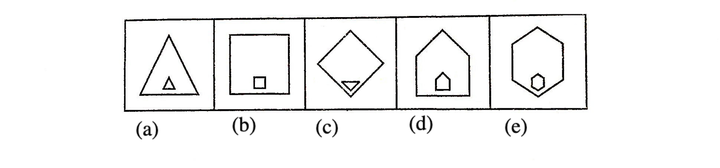Sol. (c) Except figure (c), in all the figures, both the inside and outside figures are similar but differ in size.

Example 2: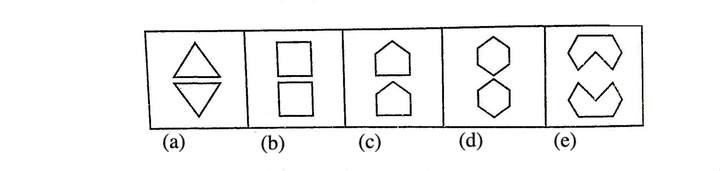Solution:  (c) Except figure (c), in all the figures are mirror images of one another.

6. Interior-Exterior Consideration of Elements

A figure can be formed from two or more elements, it is likely that some elements may lie in interior of other elements while some may lie in the exterior of the other elements. This consideration can be used for classification of elements from a group.

Directions (Example Nos. 1-2) In the following questions, a group of five figures is given, Out of which four figures are similar to each other in a certain way and one is different from other. Find the odd figure out.

Example 1: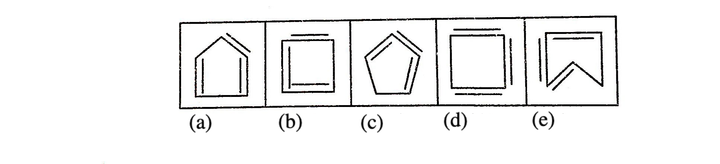Solution: (d) Only figure (d) does not contain any element present in the interior of the closed figure.

Example 2: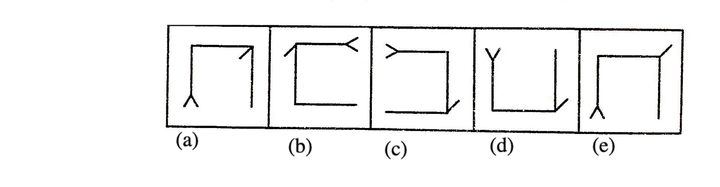Solution:  (a) In all figure, except (a) the line attached to the conner of figure is facing outside the figure but in figure (a) it is inside the figure.

MIND IT !

Based on the classification explained above, there can be two types of questions which are asked in exams.

1. Choosing the Odd Figure
2. Choosing Figure with Same Characteristics

TIPS on cracking Non Verbal Reasoning Questions on Classification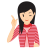Few Important things to Remember

Time is a very important thing in every exams. You should complete your exam within the time frame. But in a competitive exam, question are for testing your calculation ability within a given time frame. They tests, how quickly a student can solve a question paper. This is the reason so many students couldn’t finish their paper within given time. But if you need to solve your government or bank or any other question paper quickly then you should use tips of reasoning and Classification (Non Verbal Reasoning) which will help you to solve government, bank and other exam papers quickly.

Type - 1 : Choosing the Odd Figure

Tip# : In this type of questions, four/five figures labelled as (a), (b), (c), (d) and/or (e) are given, three/four of these four/five figures have common features/characteristics and hence are similar in a certain way. One of the figure does not share the common characteristics and hence does not fit with other figures. You have to select that to select that figure which does not belong to the group and this figure is your answer.

Following examples will give you a better idea about such type of questions:

Directions (Example Nos. 1-5) In the following questions a group of four/five figures are given. Out of which three/four figures are similar to each other in a certain pattern. Find the figure which does not belong to the group.

Example 1: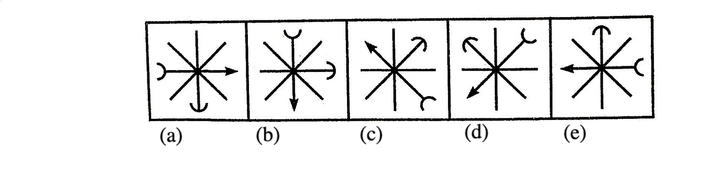Solution: (b) All are rotated forms of same figure.

Example 2: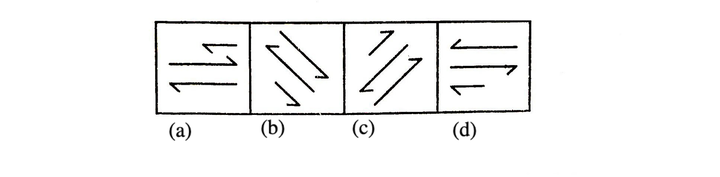Solution: (d) Two lines on the extreme ends of all the figures, except figure (d) have same direction of arrows. In figure (d), the direction of arrows are different.

Example 3: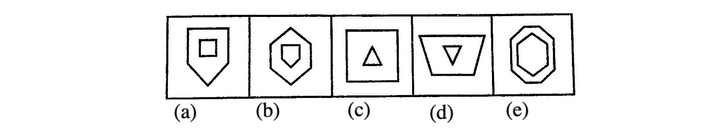Solution: (e) In all the figures, except figure (e), two concentric figures are arranged in such a way that inner figure has one side less than the outer figure.

Example 4: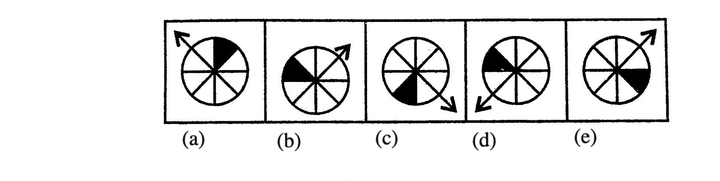Solution: (b) In all the figures, except figure (b), arrow is one step away from the shaded sector of the circle in anti-clockwise direction.

Example 5: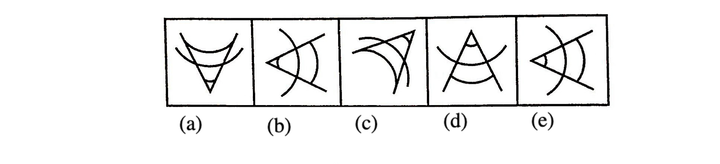Solution: (b) In all the figures, except figure (b), arcs in pair outside the hexagon are two sides ahead of the single arc.

Type - 2 : Choosing Figure with Same Characteristics

Tip# : In this type of questions, a set of problem figure and a set of answer figures are given. The problem figures are related to each other on the basis of some common properties and one of the answer figures belongs to the group of problem figures on the basis of these common properties. You are required to take out that figure which belongs to the group of problem figures and that figure is your answer.

Following examples will give you a better idea about such type of questions:

Directions (Example Nos. 1-2) In each of the following questions, there are two problem figures followed by answer figure marked as (a), (b), (c), (d) and (e). The two problem figure have some common characteristics. Select the answer figure which has same characteristics as that of the problem figure.

Example 1: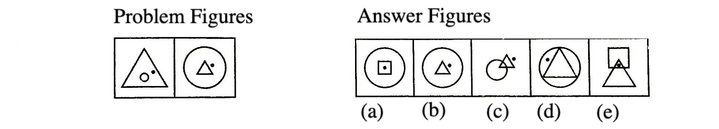Solution: (b) A dot is placed on right hand side of the inner figure.

Example 2: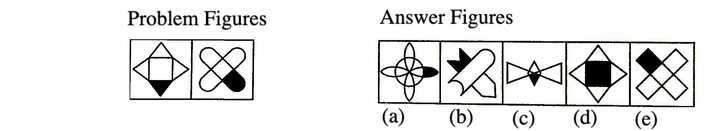Solution: (e) Two identical figures intersect each other and any one outer portion of the figure is shaded. Figure (e) has the similar characteristics and hence, belong to the same group.

Directions (Example Nos. 1-2) In each of the following questions, there is a set of four figures named as problem figures followed by answer figure marked as (a), (b), (c), (d) and (e). The four problem figure have some common characteristics. Select the answer figure which has same characteristics as that of the problem figure.

Example 1: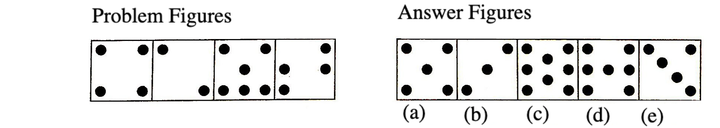Solution: (c) When we closely observe the problem figure, then we find that there are even number of dots in the problem figure. So, the answer figure having even number of dots is the correct answer i.e., option (c).

Example 2: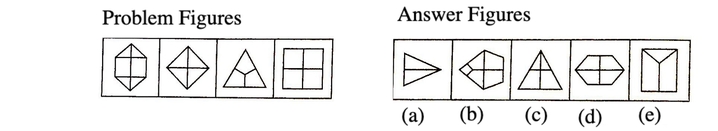Solution: (b) Each problem figure is divided into as many parts as the number of sides in it. Hence, the correct answer figure bearing the same characteristics is given in option (b).